Definitions of Square Dance Calls and Concepts

Index -->  Plus  |  A1  |  A2  |  C1  |  C2  |  C3A  |  C3B  |  C4  |  NOL  |
Definitions (Text Only) -->  Plus  |  A1  |  A2  |  C1  |  C2  |  C3A  |  C3B  |  C4  |  NOL  |
 Find call:

\$B0lHL2=\$5\$l\$?(B 1/4 Tag \$B\$^\$?\$OB>\$NE,@Z\$J(B formation \$B\$+\$i!%(B

Jay Walk \$B\$r\$9\$k?M\$?\$A\$N(B Distorted (\$B\$f\$,\$s\$@(B) Box \$B\$GF0\$-\$^\$9!%%3!<%k\$N=*\$o\$j\$G(B, \$B\$3\$l\$i\$N(B 4 \$B\$D\$N(B footprint \$B\$N0LCV\$r:F9=@.\$7\$^\$9!%(B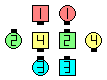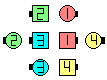Jay Left Wheel Thru\$B\$NA0(B \$B8e(B

\$B6a\$4\$m(B, \$B2??M\$+\$N%3!<%i!<\$O(B, \$B>e5-\$NL>A0\$NIU\$1J}\$r0lHL2=\$7\$F;H\$C\$F\$\$\$^\$9!%L@3N\$K(B, Jay \$B\$OJ}8~\$G<(\$5\$l(B, \$B\$=\$NJ}8~\$O(B Center Line \$B\$K\$\$\$k?M\$+\$i\$NAjBPE*\$JJ}8~\$G\$9!%\$9\$J\$o\$A(B, \$B30B&\$N?M\$N8~\$-\$K\$O4X78\$,\$"\$j\$^\$;\$s!%(B

• (Front) Jay: Center \$B\$O(B, \$B<+J,\$NA0\$K\$\$\$k(B outside \$B\$N?M\$HF0\$-\$^\$9!%(B
• Back Jay: Center \$B\$O(B, \$B<+J,\$N8e\$m\$K\$\$\$k(B outside \$B\$N?M\$HF0\$-\$^\$9!%(B
• Right | Left Jay: Center \$B\$O(B, \$B<+J,\$N1&(B | \$B:8\$K\$\$\$k(B outside \$B\$N?M\$HF0\$-\$^\$9!%(B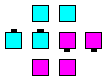(Front) Jay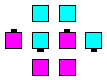Back Jay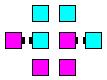Right Jay

\$BCm\$N(B formation \$B\$G\$b;H\$(\$^\$9!%\$^\$?(B, offset \$B\$K\$J\$C\$F\$\$\$k(B 2 \$B?M\$G\$b(B Jay Touch 1/4 \$B\$N\$h\$&\$J(B 2 \$B?M\$N%3!<%k\$,\$G\$-\$^\$9!%(B

Back Jay Shakedown: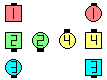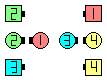Back Jay Shakedown\$B\$NA0(B \$B8e(B
Left Jay Partner Tag: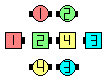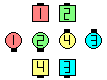Left Jay Partner Tag\$B\$NA0(B \$B8e(B

\$BNr;KE*\$K(B, Jay \$B\$O

• (Facing) Jay: \$B3F!9\$N(B distorted Box \$B\$K\$\$\$k?M\$OCf\$r8~\$\$\$F\$\$\$^\$9(B (Box \$B\$N(B Center \$B\$K8~\$+\$C\$F(B), \$B\$=\$7\$F\$*8_\$\$\$K8~\$+\$\$9g\$C\$F\$\$\$^\$9!%\$3\$l\$,I8=`\$N(B Jay \$B\$G\$9!%(B
• Back-to-Back Jay: \$B3F!9\$N(B distorted Box \$B\$K\$\$\$k?M\$O30\$r8~\$\$\$F\$\$\$^\$9(B (Box \$B\$N(B Center \$B\$+\$iN%\$l\$k\$h\$&\$K(B), \$B\$=\$7\$F\$*8_\$\$\$KGXCf9g\$o\$;\$K\$J\$C\$F\$\$\$^\$9!%(B
• Front-to-Back Jay: \$B3F!9\$N(B distorted Box \$B\$G(B Front-to-Back \$B\$K\$J\$C\$F\$\$\$F(B, \$B\$"\$k?M\$O(B Leaders \$B\$G(B, \$B\$"\$k?M\$O(B Trailers \$B\$G(B Leader \$B\$NGXCf\$r8+\$F\$\$\$^\$9!%(B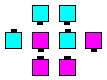Facing (normal) Jay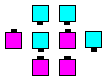Front-to-Back Jay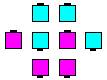Back-to-Back Jay

\$B%R%s%H(B: \$BJ#;(\$J%3!<%k\$G\$O(B, \$BF0\$-;O\$a\$kA0\$K(B Jay \$B\$N\$:\$l\$NJ}8~\$rIt20\$K\$D\$\$\$F%A%'%C%/\$7\$^\$9!%Nc\$(\$P(B, Jay \$B\$,(B offset \$B\$K\$J\$C\$F\$\$\$kJ}8~\$NJI\$r3P\$(\$F\$*\$-\$^\$9!%IaDL(B (1) Centers \$B\$O(B Two-Faced Line \$B\$J\$N\$+(B Wave \$B\$J\$N\$+\$H(B, (2) Center Line \$B\$N\$I\$A\$i\$N(B End \$B\$,Cg4V\$J\$N\$+\$r3P\$(\$F\$*\$/\$3\$H\$G(B, Jay \$B\$N0LCV\$r5-21\$7\$^\$9!%(B

\$B;~!9(B, \$B%3!<%i!<\$O(B Jay \$B\$+\$i(B Shape-changing \$B\$N%3!<%k\$r%3!<%k\$7\$^\$9!%(B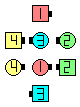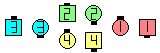Jay Pass The Ocean\$B\$NA0(B \$B8e(B

On a shape-changing call (usually from a Box to a Line or Wave), the idea is to maintain the center point between the pairs of dancers. In the above example, dancers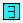and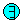finish such that their center point is the original center point between dancers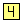and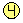. Similarly, dancersandfinish such that their center point is the original center point between dancersand(which just happens to be the original starting position of dancer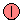).

Change Jays [NOL]: Jay \$B\$N%3!<%k\$r9T\$C\$?8e(B, \$BJL\$N(B Jay \$B\$N0LCV\$GF0\$-\$^\$9(B (Outsides \$B\$OB>\$N(B Center \$B\$N0LCV\$HF0\$-(B, Centers \$B\$OB>\$N(B Outside \$B\$N0LCV\$HF0\$-\$^\$9(B)\$B!%(B

Same Jay [NOL]: Jay \$B\$N%3!<%k\$r9T\$C\$?8e(B, \$BF1\$8(B Distorted Box (\$B\$f\$,\$s\$@(B box) \$B\$NF1\$8?M\$H(B, \$B \$B

• Jaywalk [C1].
• Jay Concept (by Charles Young)
•CALLERLAB definition for Jay ConceptChoreography for Jay ConceptComments? Questions? Suggestions?

https://www.ceder.net/def/jay.php?level=master&language=japan
07-May-2021 03:32:52